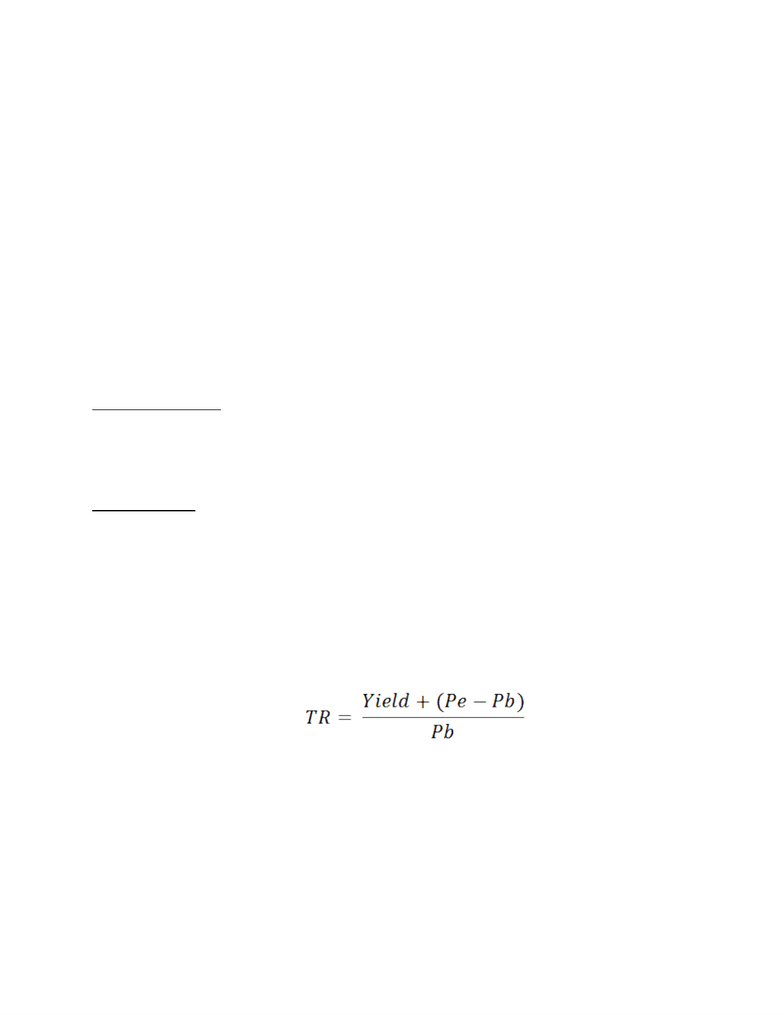Study Guides (380,000)
CA (150,000)
Ryerson (10,000)
Finance (300)
FIN 300 (100)
Quiz

# FIN 300 Study Guide - Quiz Guide: Capital Asset Pricing Model, Risk-Free Interest Rate, Equity Premium Puzzle

Department
Finance
Course Code
FIN 300
Professor
Ron Babin
Study Guide
Quiz

This preview shows pages 1-3. to view the full 9 pages of the document.Financial Securities
Chap 6, 9, & 10 Test Review
Yield (first component of Return):
Yield is the income component of a security’s return. The issuer makes this payment
in cash to the holder of the asset periodically in return for their investment.
Capital Gain/Loss (second component of Return):
Capital gain or loss is the depreciation or appreciation in a security’s price over time.
It can be measured as the difference between the sale price and the subsequent
price, if the difference is negative, then the investor is at a capital loss, if it is positive,
then they are at a capital gain.
Total Revenue Formula:
To calculate total revenue, simply add up all revenue values given.
Ex – Revenue for 2003 was \$6000, for 2004 was \$10,000, and for 2005 was \$20,000.
What is the total revenue in 2005?
Answer – 6000+10,000+20,000=\$36,000
Investing in Bonds & Shares:
Bonds have a smaller yield in the short term than shares (stocks) do; however, since
the risk in Bonds is very minimal, they typically result in a greater Capital Gain in the
long term.
The yield of shares (stocks) is determined by the risk level of the investment.
Typically, the greater the risk of the investment, the greater the yield.
Types of Risk:
SR – Systematic Risk – Risk that affects companies on a macro scale
1) Interest Rate Risk (SR) – Variability in the return of a security due to changes in
the interest rate
2) Market Risk (SR) – Variability in the return of a security due to fluctuations in
the market
3) Inflation Risk (SR) – Variability in the return of a security due to inflation, this is
closely tied with the interest rate risk as interest rates typically increase as
inflation is occurring

Only pages 1-3 are available for preview. Some parts have been intentionally blurred.4) Exchange Rate Risk (SR) – Variability in the return of a security due to currency
fluctuations, international investors are the most who are affected by this
5) Country Risk (SR) – Variability in the success of companies that deal
internationally as a result of political or economic turmoil in the country
wherein which the company exists
NSR – Non-Systematic Risk – Risk that affects specific & individual companies
1) Business Risk (NSR) – The risk of doing business in only one specific industry
2) Financial Risk (NSR) – The risk in having a company financed mostly by debt,
which results in much lower returns (Companies can’t pay shareholders if they
can’t pay creditors)
3) Liquidity Risk (NSR) – The risk of company’s inability to be liquid (bought and
sold) in the stock market, thus leading to no change in the value of stock
Risk Prevention:
Risk cannot be prevented, but it can be controlled. Risk control is done through beta
calculations, and the CAPM formula. Another way to control risk is to diversify your
investments.
Total Return:
- Total return is the percentage measure relating all cash flows on a security for
a given time period to its purchase price
- 2 components of TR are yield & price change
- If ending price of stock is > beginning price, and dividends are low, TR is
negative
- TR is calculated with this formula,
- Where,
TR = Total Return
Yield = Dividend paid by company
Pe = The most recent price of the stock
Pb = The paid price of the stock

Only pages 1-3 are available for preview. Some parts have been intentionally blurred.CAPM:
The Capital Asset Pricing Model (CAPM) relates the required rate of return for any
security with the market risk for that security as measured by beta. Since CAPM is
only an estimation, there are 8 assumptions that are always made when calculating
CAPM so as to maintain consistency in results
1) All investors have identical probability distributions for future rates of return
2) All investors have the same one-period time horizon
3) All investors can borrow or lend money at the risk-free rate of return
4) There are no transaction costs
5) There are no personal income taxes
6) There is no inflation
7) No single investor can affect the price of stock through his buying/selling
decisions.
8) Capital markets are in equilibrium
The formula to calculate CAPM’s required rate of return is as follows:
K(i) =RF + B(i) [ E(Rm) –RF]
Where,
K (i) = required rate of return of investment ‘i’
RF = risk free rate of return
B(i) = Beta coefficient of investment ‘i’
E(Rm) = Expected rate of return on the market portfolio
Beware that in many cases, the expected rate of return is not given and must be
calculated by using this formula (or variations of it based on the data given):
E(Rm) = E(Rp) + RF
Where,
E(Rm) = Expected rate of return on the market portfolio
E(Rp) = Equity risk premium
###### You're Reading a Preview

Unlock to view full version